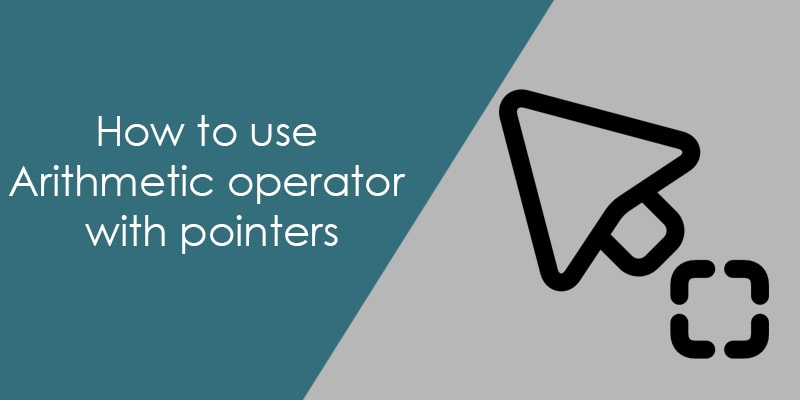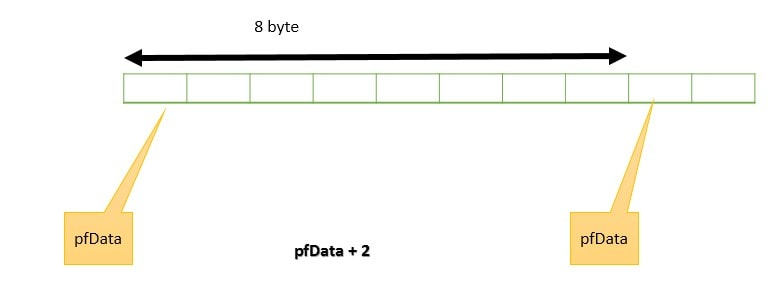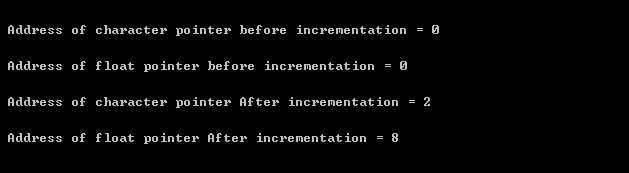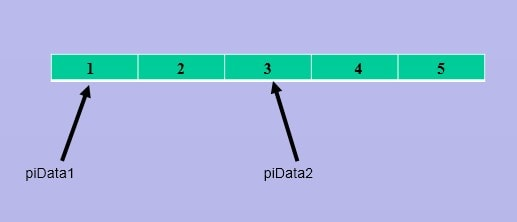# How to use arithmetic operator with pointersIn program sometimes we need to perform some pointer arithmetic operation, but before using any arithmetic operation on pointer we need to understand the limitation of the arithmetic operator with pointers.

The C language provides a lot of arithmetic operators but generally, with the pointer, we use increment (++) or decrement (–) operator to increment or decrement the pointer. There is also a way to increment or decrement the pointer to add or subtract an integer number from the pointer using the plus (+) or minus (-) operator.

When we have performed the arithmetic operation on pointer then we need to remember that we cannot use the multiplication or division operator with the pointer.

##### I have listed some arithmetic operators in the below list
 Indirection (*) This operator is used to get the value from the pointed address. Reference operator( &) This operator is used get the address of the variable or pointer. Assignment ( =) You can assign the value to the pointer or value to the address which is pointed by the pointer. Incrementing You can add integer value to the pointer to point the different memory location. Decrementing You can subtract the integer value from the pointer to point the different memory location. comparison This operation is valid only between two pointers that point to the same array.

Note: When we increment or decrement the pointer then pointer increase or decrease a block of memory (block of memory depends on pointer data type).

### How does pointer arithmetic work

When we increment or decrement the pointer then pointer point to the next or previous memory location. Generally, people make mistakes, when they calculate the next pointing address of the pointer.

Here is the technique for computing the next pointing address of pointer when we have performed an arithmetic operation on the pointer.

where ptr is a pointer has data type T and i is the pointer offset.

addr( ptr + i ) =  addr( ptr ) + ( sizeof( T ) * i );

addr( ptr – i ) =  addr( ptr ) – ( sizeof( T ) * i );

So for the character pointer when we increment pointer then it incremented 1 byte of the address. If we increment the float pointer then it increments 4 bytes of address.

To understand pointer arithmetic, Let us consider that pcData is a character pointer.

char *pcData =NULL;

When we increment the pcData then it points to the next character location without impacting the stored data. The size of the character is 1 byte that’s why pointer moves only 1 byte of memory.

pcData++;

In this scenario, pcData is pointer to character. So the address of pcData + 1 is:

addr( pcData + 1 ) = addr( pcData ) + [ sizeof( char ) * 1 ];
addr( pcData + 1 ) = addr( pcData ) + [ 1 * 1 ];
addr( pcData + 1 ) = addr( pcData ) + 1;Like the character pointer, when we increment the float pointer then it points to next float location without impacting the stored data.

float *pfData = NULL;

pfData++;

In this scenario, pfData is pointer to float. So the address of pfData + 1 is:

addr( pfData + 1 ) = addr( pfData ) + [ sizeof( float) * 1 ];
addr( pfData + 1 ) = addr( pfData ) + [ 4 * 1 ];
addr( pfData + 1 ) = addr( pfData ) + 4;### Program to increment a pointer in c

In below program, I am creating a character and float pointer and incrementing the pointer by 1 using the increment and decrement operator.After performing the arithmetic operation on pointer I am printing their value to see the effect of pointer arithmetic.If on the other hand, we added 2 to the pcData (character pointer ) then it moves the pcData to the 2 character positions (2 bytes).
char *pcData = NULL;

pcData = pcData + 2;Same like the character pointer, if we added 2 to the pfData( float pointer) then it moves pfData 2 float position (8 bytes).

float *pfData = NULL;

pfData = pfData + 2;### Pointer subtraction in C

In C language, we can also subtract one pointer from the other pointer. This technique is useful when we need to calculate the number of bytes between the two pointers. But we subtract two pointers only when they pointing the same array or memory block.The result of this subtraction gives the number of elements that separating them

Note: There are is no meaning to the addition of two pointers.

Let’s consider two example which describes the subtraction of two number.

Example 1:
In this example, we will calculate the size of the structure to subtract the pointers.

Example 2:
In this example, we will calculate the number of elements that separating the pointers or you can say calculate the pointer offset.### Pointer comparison in C

Like the pointer subtraction, we can also compare the pointer but pointer should be pointed to the same array or memory block.
See the below example;

char acBuffer = “aticleworld”;
char *pc1, *pc2;

Assigning address of first  and third elements of acBuffer1 to pc1 and pc2.

pc1 = &acBuffer; // Address of first element

pc2 = &acBuffer; // Address of third element

Now we can compare the pointers if required.

if( pc1 > pc2) // Legal statement
{

}

But pointers comparison is invalid when pointers point to the 2 different memory blocks.

See the below example;

char acBuffer1 = “aticle”;
char acBuffer2 = “world”;
char *pc1, *pc2;

Assining the address of acBuffer1 to pc1 and acBuffer2 to the pc2.

pc1 = acBuffer1;
pc2 = acBuffer2;

In that situation pointer comparison is invalid.
if( pc1 > pc2) // illegal statement
{
}

Note: The C standard does not allow the arithmetic operation on void pointers but GNU C allows with assuming the size of the void is 1. To know about the void pointer See this Link: Use of void pointer in C language.